# Formula Auditing in Microsoft Excel: How to Track a Formula’s Calculations

## Formula Auditing Tools

Problem: You are trying to trace how a formula is calculating. If you have Excel 2002 or a later version, there is a cool new tool on the Formula Auditing menu. (The Formula Auditing tools are found on the Formulas tab in Excel 2007 and later.)

If you’re not getting the type of answer that you’d expect from a formula, this feature can help track the problem down. It’s also a nice tool to use when you’re trying to understand a spreadsheet exhibit that has been created by someone else.

## How is a Formula Being Calculated?

Strategy: Select the cell with the formula. From the menu, select Tools – Formula Auditing – Evaluate Formula, as shown in Fig. 484. (Click any image for a larger view.)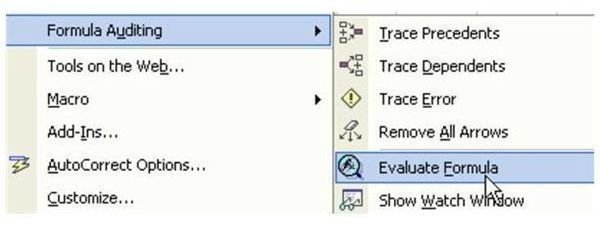Note: In Excel 2007, the Evaluate Formula tool is found in the Formula Auditing grouping on the Formulas tab.

The Evaluate Formula dialog shows the formula. The first item to be calculated is underlined, as shown in Fig. 485. Click Evaluate to calculate the underlined portion of the formula.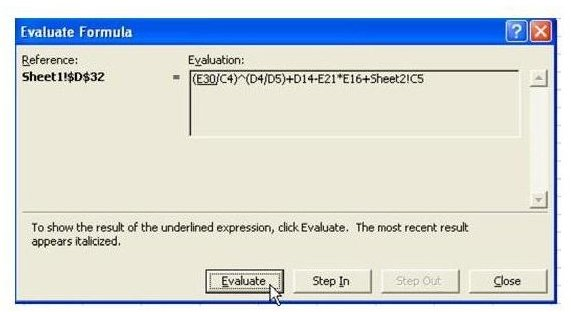With each click of Evaluate, Excel will calculate the underlined portion and show the results in italics. It will underline the next step in the calculation. Fig. 486 is after the second Evaluate. Excel just revealed that C4 is 1200 (in italics). It is about to calculate the first division in parentheses.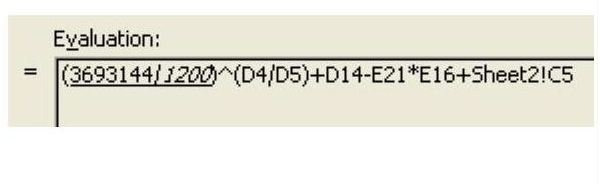Additional Information: Any time that the next term to be calculated is a cell reference, you can choose the Step In button to evaluate the formula in that cell. In Fig. 487, choosing Step In will evaluate D14.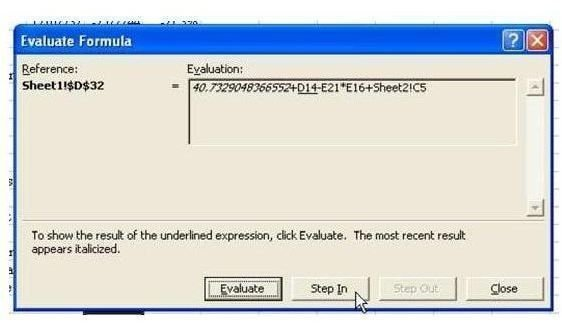As shown in Fig. 488, it is possible to Step In several levels. After seeing the formula for D14, you can choose to Step In to see the formula for D15.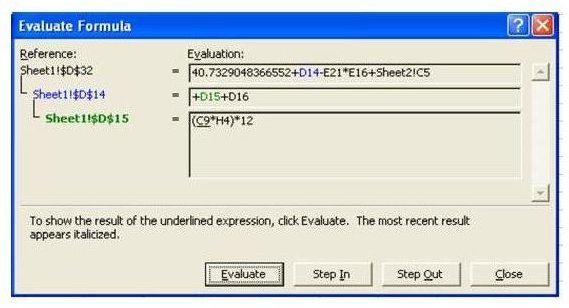Use Step Out to close the most recent detail level and go back one level, as shown in Fig. 489.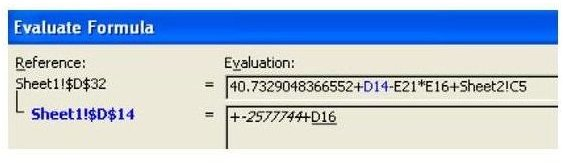Summary: This feature is a great tool. It basically gives you a great appreciation of just how much work Excel does every time you enter a formula, because it allows you to watch the calculation happen in slow motion.

Commands Discussed: Tools – Formula Auditing – Evaluate Formula

## More Excel Tips

If you’re interested in more Excel tips, be sure to check out the hundreds of other Microsoft Excel tutorials available at Bright Hub. In particular, you may find the following collections useful.

91 Tips for Calculating with Microsoft Excel – This set of easy-to-follow tutorials explores Excel’s calculating abilities from every angles. Learn more about how formulas and functions work, discover shortcuts for typing cell references, and more.

Excel Formatting Tips from Mr. Excel – Focusing on Excel’s formatting capabilities, this collection of 72 tips offers advice on how to better organize and analyze spreadsheet data, while making it look good in the process.